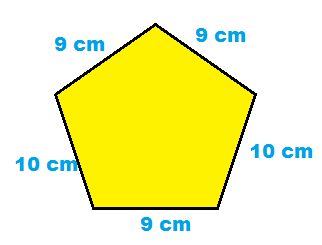# Perimeter Lesson for Kids: Definition & Examples

Instructions:

question 1 of 3

### What does perimeter measure?

Create Your Account To Take This Quiz

As a member, you'll also get unlimited access to over 79,000 lessons in math, English, science, history, and more. Plus, get practice tests, quizzes, and personalized coaching to help you succeed.

Try it risk-free for 30 days. Cancel anytime

### 1. Find the perimeter of the pentagon.### 2. The playground is a rectangle. It is 45 yards long and 33 yards wide. What is the playground's perimeter?

Create your account to access this entire worksheet
Quizzes, practice exams & worksheets
Certificate of Completion
Create an account to get started

Look at the quiz and worksheet to check your understanding of perimeter. You will need to be able to find the perimeter in different examples for the quiz.

## Quiz & Worksheet Goals

• A shape that has a perimeter of 90 feet
• What perimeter measures
• Finding the perimeter of different shapes

## Skills Practice

Use the multiple-choice quiz to practice the following skills:

• Reading comprehension - make sure that you pick out the most important details from the math lesson on perimeter
• Information recall - remember the information you gained about what perimeter measures
• Problem solving - use what you've learned to solve perimeter practice problems

Practice finding the perimeter a little bit more by using the lesson Perimeter Lesson for Kids: Definition & Examples. Thanks to this lesson, you can:

• Understand why perimeter is used
• Look at different examples of perimeter being used
• Figure out how to find the perimeter of different shapes
Final ExamMath for Kids
Status: Not Started
Chapter ExamGeometry for Elementary School
Status: Not Started

Support